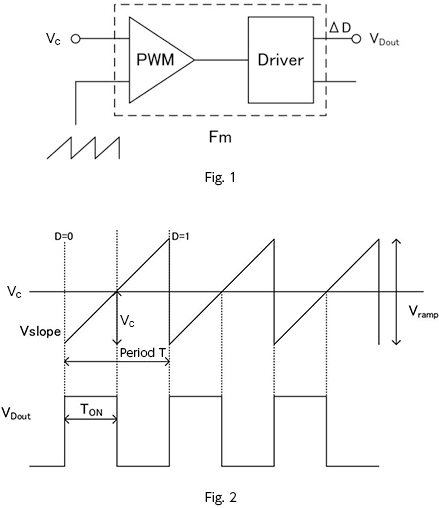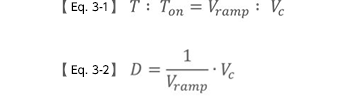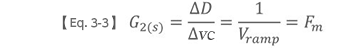# Voltage-Mode Transfer Functions

2017.01.12

・In this section, we first consider transfer functions for voltage-mode control.## Voltage-mode transfer functions

Voltage-mode control in a DC-DC converter is the most basic system. Only the output voltage is fed back through a feedback loop. By using the voltage difference obtained by comparison with a reference voltage using an error amplifier, and further comparing with a slope wave (triangular wave), the pulse width of a PWM signal is determined and the output voltage is controlled.

Fig. 1 shows a block in which the output Vc from the error amplifier and the slope voltage are input to the PWM generator to generate the PWM output.

Here, by comparing a fixed slope wave with Vc, the transfer function of the block Fm, which is PWM controlling the duty D = Ton/T, is derived.

Fig. 2 shows the input waveform and the PWM output waveform. The slope wave rises with a constant slope by Vramp over one period. Through comparison with Vc, the on and off times of the driver output VDout, that is, the duty cycle, is determined. The control conditions are as follows.

When Vc ＞ Vslope , VDout = ON
When Vc ＜ Vslope , VDout = OFF

Moreover, in Fig. 2:

When Vslope = Vc = ０  ,    D = 0
When Vslope = Vc = Vramp , D = 1

Equation 3-1 is obtained from similarity of the triangle formed from Vslope and Vc, and by modifying this equation using D = Ton/T, we obtain equation 3-2.Moreover, from perturbation of equation 3-2 （ D → D + ?D , Vc → Vc + ?VC） equation 3-3 is obtained.